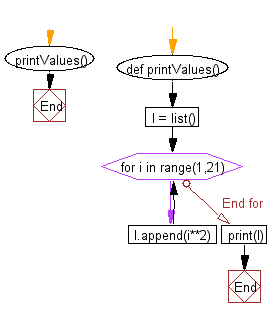﻿ Python Exercise: Generate and print a list where the values are square of numbers between two numbers - w3resource# Python Exercise: Generate and print a list where the values are square of numbers between two numbers

## Python Functions: Exercise - 16 with Solution

Write a Python function to create and print a list where the values are square of numbers between 1 and 30 (both included).

Sample Solution:-

Python Code:

``````def printValues():
l = list()
for i in range(1,21):
l.append(i**2)
print(l)

printValues()
```
```

Sample Output:

```[1, 4, 9, 16, 25, 36, 49, 64, 81, 100, 121, 144, 169, 196, 225, 256, 289, 324, 361, 400]
```

Pictorial presentation:Flowchart:## Visualize Python code execution:

The following tool visualize what the computer is doing step-by-step as it executes the said program:

Python Code Editor:

Have another way to solve this solution? Contribute your code (and comments) through Disqus.

What is the difficulty level of this exercise?

Test your Programming skills with w3resource's quiz.

﻿

## Python: Tips of the Day

Decapitalizes the first letter of a string:

Example:

```def tips_decapitalize(s, upper_rest=False):
return s[:1].lower() + (s[1:].upper() if upper_rest else s[1:])
print(tips_decapitalize('PythonTips'))
print(tips_decapitalize('PythonTips', True))
```

Output:

```pythonTips
pYTHONTIPS
```